# SAT II Physics : Electricity

## Example Questions

### Example Question #1 : Ohm's Law

If a circuit contains a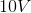battery and a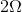resistor what is the current of the circuit?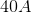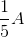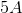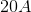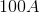Explanation:

Ohm's law states: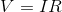Whereis voltage,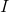is current, andis resistance.

We can substitute the given values from the question into the equation and solve: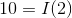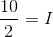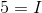### Example Question #1 : Electricity

There are three resistors in parallel in a circuit with resistances of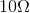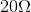, and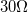.

What is the equivalent resistance?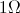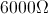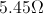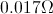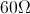Explanation:

The equation for resistors in parallel is: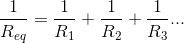We are given the values of the resistors. Using this formula, we can solve for the equivalent resistance.

Plug in the given values and solve.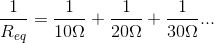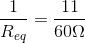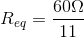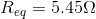### Example Question #1 : Electricity

A voltage ofapplied between the ends of a wire results in a current of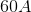. What is the resistance of the wire?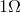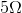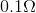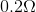Explanation:

This question can be solved using Ohm's law:We are given the voltage and the current. Using these values, we can solve for the resistance.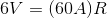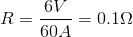### Example Question #1 : Electricity And Magnetism

A capacitor with capacitanceis constructed by putting a thin piece of cardboard between two copper plates, then each plate is connected to a battery. If the copper plates are cut in half then what is the effect on the capacitance of the circuit?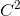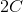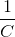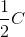Explanation:

Capacitance of a circuit is defined by the equation: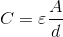Whereis the capacitance,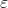is a constant of nature,is the area of the capacitor, andis the distance between the two plates

Since the metal plates are cut in half, the area is halved. We can substitute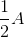in forThe result is that the capacitance is half the original quantity.

### Example Question #1 : Coulomb's Law

If the distance between two charged particles is doubled, the strength of the electric force between them will __________.

be halved

be quartered

double

remain unchanged

be quartered

Explanation:

Coulomb's law gives the relationship between the force of an electric field and the distance between two charges: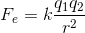The strength of the force will be inversely proportional to the square of the distance between the charges.

When the distance between the charges is doubled, the total force will be divided by four (quartered).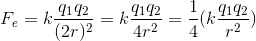### Example Question #1 : Electricity And Magnetism

On which of the following does the amount of work required to move a charge in an electric field depend?

Only the change in potential

Only the magnetic field

Both the potential and the path traveled

Neither the potential nor the path traveled

Only the path traveled

Only the change in potential

Explanation:

Work done by electric field is defined: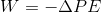Notice that the only variable in the equation is the potential, so this is the only quantity on which work depends.

### All SAT II Physics Resources